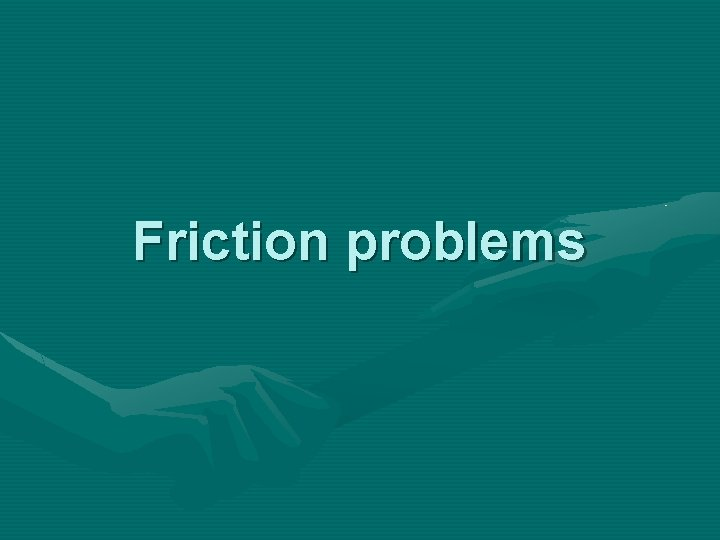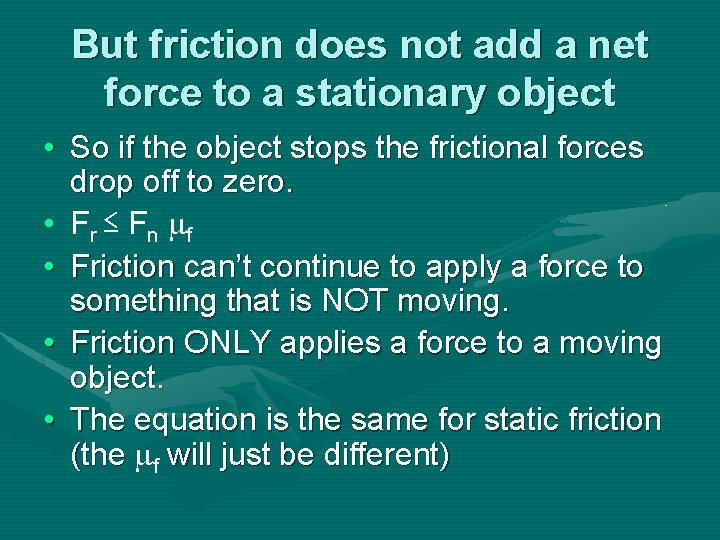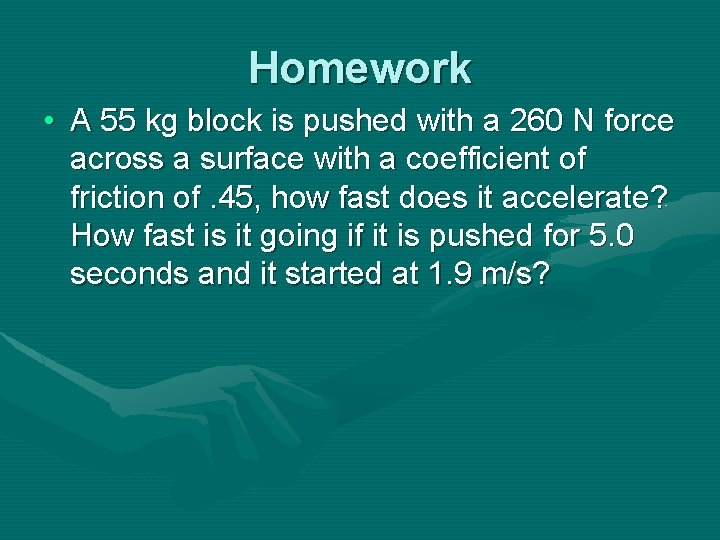# Friction problems Sliding Friction Sliding Friction is determined

• Slides: 6Friction problemsSliding Friction • Sliding Friction is determined by: The Force normal of the ground (reaction to the object’s weight) ~this will equal the weight if the object isn’t moving up or down And how hard the object resists you moving against it, the coefficient of friction ( f) Fn= mg (if you are not moving up or down) f So… F r= F n fBut friction does not add a net force to a stationary object • So if the object stops the frictional forces drop off to zero. • F r ≤ F n f • Friction can’t continue to apply a force to something that is NOT moving. • Friction ONLY applies a force to a moving object. • The equation is the same for static friction (the f will just be different)Problem • A 35 kg block is pushed with a 210 N force across a surface with a coefficient of friction of. 35, how fast does it accelerate? How fast will it be going after 3. 0 seconds if it starts at 1. 5 m/s • Frictional force F r= F n f • Fr = 35 kg (9. 8 m/s 2). 35 = 120. 05 N • ΣF = 210 N – 120. 05 = 89. 95 N • Σ F = ma • 89. 95 N= 35 kg (a) • a = 2. 6 m/s 2 • a= (vf – vi)/t • 2. 6 = (v – 1. 5 m/s)/3 s v = 9. 3 m/sProblem • A 45 kg block is pushed with a 165 N force across a surface with a coefficient of friction of. 45, how fast does it accelerate? How fast is it going if it started at 1. 5 m/s and went for 3. 0 seconds? • Frictional force F r= F n f • Fr = 45 kg (9. 8 m/s 2). 45 = 198. 45 N • ΣF = 165 N – 198. 45 = -33 N Friction can’t reduce it’s • Σ F = ma velocity to less than 0. So the • 33. 45 N= 45 kg (a) object will be at rest, 0 m/s • a = -0. 74 m/s 2 • a= (vf – vi)/tHomework • A 55 kg block is pushed with a 260 N force across a surface with a coefficient of friction of. 45, how fast does it accelerate? How fast is it going if it is pushed for 5. 0 seconds and it started at 1. 9 m/s?